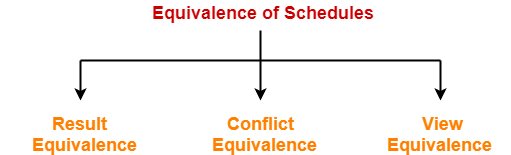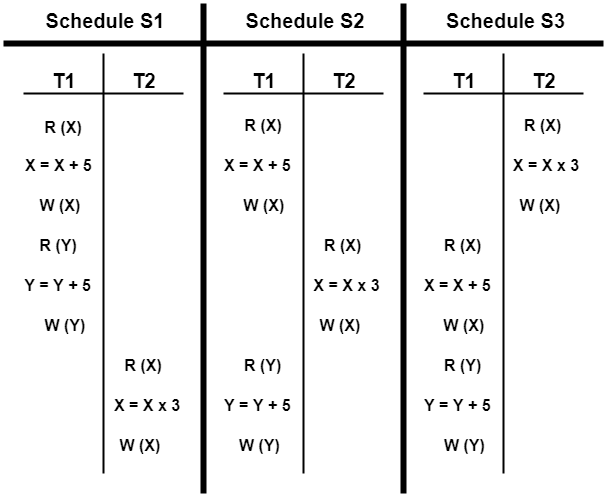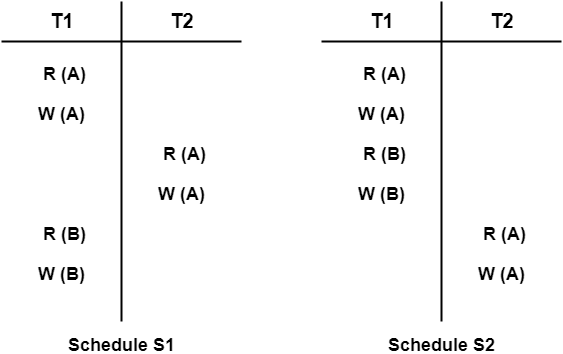## Equivalence of Schedules-

In DBMS, schedules may have the following three different kinds of equivalence relations among them-1. Result Equivalence
2. Conflict Equivalence
3. View Equivalence

## 1. Result Equivalent Schedules-

• If any two schedules generate the same result after their execution, then they are called as result equivalent schedules.
• This equivalence relation is considered of least significance.
• This is because some schedules might produce same results for some set of values and different results for some other set of values.

## 2. Conflict Equivalent Schedules-

If any two schedules satisfy the following two conditions, then they are called as conflict equivalent schedules-

1. The set of transactions present in both the schedules is same.
2. The order of pairs of conflicting operations of both the schedules is same.

## Problem-01:

Are the following three schedules result equivalent?## Solution-

To check whether the given schedules are result equivalent or not,

• We will consider some arbitrary values of X and Y.
• Then, we will compare the results produced by each schedule.
• Those schedules which produce the same results will be result equivalent.

Let X = 2 and Y = 5.

On substituting these values, the results produced by each schedule are-

Results by Schedule S1- X = 21 and Y = 10

Results by Schedule S2- X = 21 and Y = 10

Results by Schedule S3- X = 11 and Y = 10

• Clearly, the results produced by schedules S1 and S2 are same.
• Thus, we conclude that S1 and S2 are result equivalent schedules.

## Problem-02:

Are the following two schedules conflict equivalent?## Solution-

To check whether the given schedules are conflict equivalent or not,

• We will write their order of pairs of conflicting operations.
• Then, we will compare the order of both the schedules.
• If both the schedules are found to have the same order, then they will be conflict equivalent.

### For schedule S1-

The required order is-

• R1(A) , W2(A)
• W1(A) , R2(A)
• W1(A) , W2(A)

### For schedule S2-

The required order is-

• R1(A) , W2(A)
• W1(A) , R2(A)
• W1(A) , W2(A)

• Clearly, both the given schedules have the same order.
• Thus, we conclude that S1 and S2 are conflict equivalent schedules.

Next Article- Introduction to Relational Algebra

Get more notes and other study material of Database Management System (DBMS).

Watch video lectures by visiting our YouTube channel LearnVidFun.